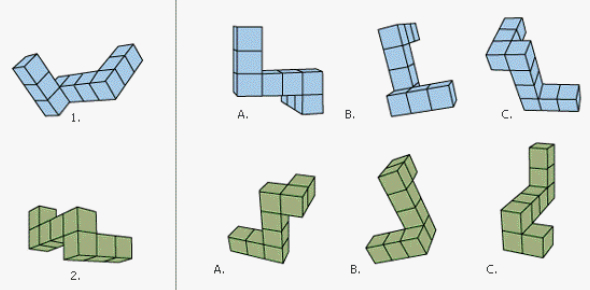# Mental Rotation Quiz Questions

10 Questions | Total Attempts: 19463SettingsThis task requires the participant to choose the figure which is identical to the original figure, aside from its orientation.

• 1.
Which image is the same as the original image, aside from its orientation?
• A.

Figure A

• B.

Figure B

• C.

Figure C

• D.

Figure D

• 2.
Which image is the same as the original image, aside from its orientation?
• A.

Figure A

• B.

Figure B

• C.

Figure C

• D.

Figure D

• 3.
Which image is the same as the original image, aside from its orientation?
• A.

Figure A

• B.

Figure B

• C.

Figure C

• D.

Figure D

• 4.
Which image is the same as the original image, aside from its orientation?
• A.

Figure A

• B.

Figure B

• C.

Figure C

• D.

Figure D

• 5.
Which image is the same as the original image, aside from its orientation?
• A.

Figure A

• B.

Figure B

• C.

Figure C

• D.

Figure D

• 6.
Which image is the same as the original image, aside from its orientation?
• A.

Figure A

• B.

Figure B

• C.

Figure C

• D.

Figure D

• 7.
Which image is the same as the original image, aside from its orientation?
• A.

Figure A

• B.

Figure B

• C.

Figure C

• D.

Figure D

• 8.
Which image is the same as the original image, aside from its orientation?
• A.

Figure A

• B.

Figure B

• C.

Figure C

• D.

Figure D

• 9.
Which image is the same as the original image, aside from its orientation?
• A.

Figure A

• B.

Figure B

• C.

Figure C

• D.

Figure D

• 10.
Which image is the same as the original image, aside from its orientation?
• A.

Figure A

• B.

Figure B

• C.

Figure C

• D.

Figure D

Related TopicsBack to top